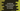# Count specific character occurrence in a string in Javascript## Introduction :

In this tutorial, we will learn how to count the occurrence of a character in a string in Javascript. We will show you how to do this using two different ways. You can save the program in a javascript .js file and verify it by running the file using node. Or, you can create one simple HTML file and test the code.

### Method 1: Using a loop :

This is the most straight-forward way to find out the total character occurrence. We can use one for loop or one while loop. The main concept is same

### Using a for loop :

The length of a string can be find out by reading the .length property in Javascript. We can also get the character at any position of a string using charAt method. str.charAt(i) returns the character at index i for the string str. The index starts at 0 for string characters. Using a for loop, we can iterate through the characters of that string one by one and�compare each with the given character. Using a counter variable, the total occurrence count can be found easily.

Complete program :

``````    let givenStr = "Hello world !!";

let ch = 'l';
let count = 0;

for(let i = 0; i<givenStr.length; i++){
if(givenStr.charAt(i) == ch){
count ++;
}
}

console.log(`Total occurrence : \${count}`);
``````

Here, we are finding the total occurrence of l in the string givenStr. If you run this program, it will print 3 as the output.

### Method 2: Using a while loop :

Similar to a for loop, we can also use one while loop to find out the total occurrence of a character in a string. Let me show you how :

``````    let givenStr = "Hello world !!";

let ch = '!';
let count = 0;
let i = givenStr.length - 1;

while(i >= 0){
if(givenStr.charAt(i) == ch){
count ++;
}
i--;
}

console.log(`Total occurrence : \${count}`);
``````

Here, we have initialized the variable i as the last character index of the string. Inside the loop, we are performing the same as we did in the for loop. The only difference is that it compares all characters from end to start and the for loop compares from start to end.

### Using Javascript string indexOf() method :

indexOf is an useful method to find the index of any substring in Javascript string. Using this method, we can determine if a string contains one substring. It is defined as below :

``````    str.indexOf(s[, i])
``````

Here, s is the substring to search in the string str. i is an optional value that represents the starting index for the search. Its value is 0 by default.

It returns the first occurrence index of the substring. If the substring is not found, it returns -1.

In our example, we will find the first index of the character in the string and starting from that index, we will find if the character . Let’s have a look :

``````    let givenStr = "Hello world !!";
let count = 0;

let p = givenStr.indexOf('!');

while (p !== -1) {
count++;
p = givenStr.indexOf('!', p + 1);
}

console.log(`Total occurrence : \${count}`);
``````

It picked the first index of the character and starting from that index, it started to find the other occurrence indices.

### Conclusion :

In this tutorial, we have learned different ways to find the total occurrence of a character in a string. Note that all of these methods are case sensitive. You can convert both character and string to lowercase at the start of the program to check for any case characters.

Try to run the programs and drop one comment below if you have any queries.